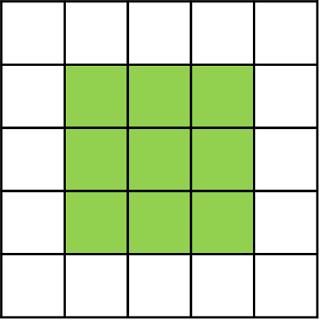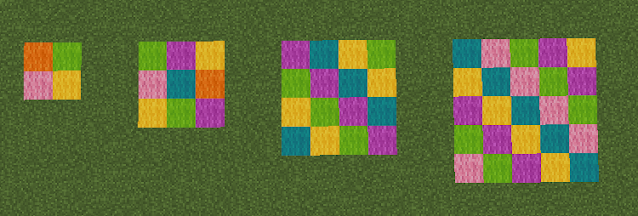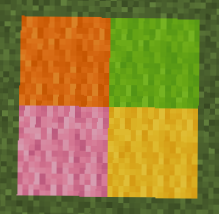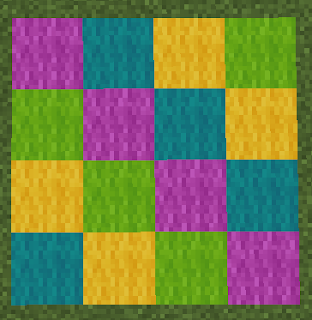## Pages

### Minecraft Math Find the Area

With Minecraft being the rage among children... and adults... of all ages, teachers are wondering how they can tap into the excitement and use Minecraft as a teaching tool. Believe it or not, Minecraft can be used to teach math skills such as finding the area, volume, and perimeter of shapes.

For the purpose of this blog post, I’m not going to teach you how to play Minecraft. There are many resources online and books you can read to learn how to play it. Rather, I’m going to show you how to use Minecraft to introduce finding the area to your young students.

Disclosure: Affiliate links to Amazon are included in this post.

## How to Find the Area of a Square

When students are asked to find the area of a shape, they need to figure out how much space is taken up by a two-dimensional (2-D or flat) shape or surface. There are several strategies that can be used for each kind of shape, but today's blog post will be focusing only on finding the area for squares and rectangles.## 1. Find the area by counting the units.

Students start learning how to find the area of a shape as early as kindergarten and first grade.  At this stage, they are asked to find the area by simply counting the number of units in a shape.

Earlier this week I made a map in Minecraft and added these five squares. One block in the square represents one unit. These examples are similar to what you’d find in a math book. Count the number of blocks in each shape. What is the area of each one? Keep reading for the answers!## 2. Find the area by multiplying length x width.

When the students get to second and third grade, they need to apply their multiplication skills to find the area of squares and rectangles. They learn that area = length x width

Take a look at each shape in the first photo again. Count the number of squares across the bottom... that is the length. Count the number of squares going up the side... that is the width. Multiply the two numbers together to find the area. Were you able to figure out the area for each shape?

## What is the area of these squares?

Here are the areas of each square. Remember... the area is always measured in square units.The area of this square is one square unit.The area of this square is four square units.The area of this square is nine square units.The area of this square is 16 square units.The area of this square is 25 square units.